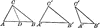# Proof of Equal Triangles DrawingIllustration to show if two triangles have two sides of the one equal, respectively, to two sides of the other, and the angles opposite two equal sides equal, the angles opposite the other two equal sides are equal or supplementary, and if equal the triangles are equal.

### Keywords

triangle, equal, angle, side, triangles, angles, Sides

### Galleries

Miscellaneous Triangles

### Source

G.A. Wentworth Plane And Solid Geometry (Boston: Ginn & Company, 1899) 66

TIFF (full resolution)

2400×677, 98.3 KiB

Large GIF

1024×288, 14.6 KiB

Medium GIF

640×180, 8.7 KiB

Small GIF

320×90, 4.2 KiB